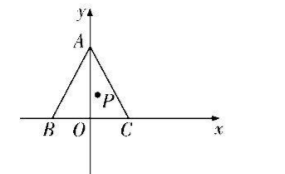【答案】 $\sqrt{7}$

【解析】 建立如图所示坐标系, 其中 $O$ 为 $B C$ 的中点, 所以 $A(0,3 \sqrt{3}), B(-3,0), C(3,0)$.$(-3 x-6-2 x+3-x, 9 \sqrt{3}-3 y-2 y-y)=0$, 即 $-6 x-3=0,9 \sqrt{3}-6 y=0$, 所以 $P\left(-\frac{1}{2}, \frac{3 \sqrt{3}}{2}\right)$,